P R O F I L EG A L L E R YO R D E RC O N T A C T    I N F OH  U  M  A  N     S  H  A  P  E    &    F  O  R  M

"There is something timeless and base about the human body.
When I am lost, I can always return to it and feel at home."

G A L L E R Y
F E A T U R E D   E X H I B I T
L A N D S C A P E
P E O P L E   &   L I F E S T Y L E

> H U M A N   S H A P E   &   F O R M
S T I L L   L I F E
E X P E R I M E N T A L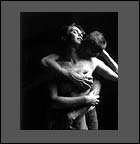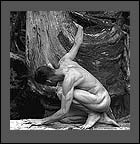c a n   y o u   f e e l   h e r ?u n t i t l e da n i m a l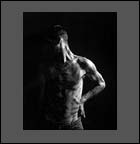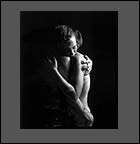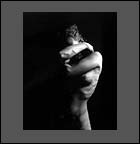a l m o s ty o u   A R E   t h e   o n en o t   y e t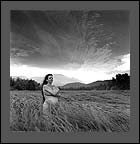k i r s t yd e n i s e   a n d   r i l e yh e s i t a t e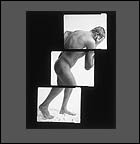u n t i t l e d >  S E N D   T H I S   S I T E   T O   A   F R I E N DT O P© 2001 - 2005   R o b e r  t   J   W i l k i n s o n             P r i v a c y   P o l i c ys i t e   d e s i g n